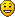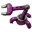## Instruction pipeline computer & sieve of Eratosthenes

This board is to show, discuss and archive useful combinator- and logic-creations.
Smart triggering, counters and sensors, useful circuitry, switching as an art, computers.
XKnight
Filter InserterPosts: 329
Joined: Thu May 28, 2015 10:40 pm
Contact:

### Instruction pipeline computer & sieve of Eratosthenes

Hi guys,

Recently several people had shared their combinator-based computers, now it is my turn:)
The main goal was to create easily-extendeble CPU (probably with multithreading, but i am still working on this) with hig-performance and very flexible to adding/removing/changing its functionality.

Stage 0: "The beginning"
This setup contains only vital things of any CPU: IP (instruction pointer) with increment, several registers, ROM memory (2 cell) and 2 instructions, *RAM memory is not vital for computer.
On this simple CPU we've already can write simple program, lets do it.
Our program will be very small and simple because our CPU is almost useless.

Code: Select all

``````start:
r0 = r0 + 1
jmp start
``````stage0.png (344.68 KiB) Viewed 4784 times
Stage 1: Peripheral devices
At this moment I don't know all purposes for this CPU, but I am going to show how to use it by yourself.
Each peripheral devices requires its own system command to communicate with this device.
If you will be able to write your own command than you will be able to use any devices you want.

Code: Select all

``````start:
r0 = r0 + 1
print r0
jmp start
``````
Here I've added one new instruction to communicate with digit display, this instruction transforms our R0 to accepted interface between CPU an device. In my example it is just transforming R0 to "blue signal", but this protocol can be complex in some cases.stage1.png (1.25 MiB) Viewed 4784 times
Stage 2: "First program"
The main idea of this CPU is to implement only necessary instructions, and reuse them in other purposes when it is possible. New instruction appears from real applications, this is the reason why I am describing this CPU together with code. On the previous stage we've implemented simple counter from 1 to infinity (2148M). Now our task will be more complex: our counter will go from 1 upto 100 and from 100 downto 1, and again from the begining.

Code: Select all

``````                  r1 = -1
increase_init:    r1 = r1 + 2
r2 = r2 + 100
increase:         r0 = r0 + r1
print r0
if (r0 == r2)  jmp decrease_init
jmp increase

decrease_init:    r1 = r1 - 2
r2 = r2 - 100
decrease:         r0 = r0 + r1
print r0
if (r0 == r2) jmp increase_init
jmp decrease
``````
Lets look on this code more deeply. At first we need to identify instructions that we already have:
1: basic instruction: register + constant
2: print r0 to display
Not so much as you might think:) After that we need to identify instructions that we need to implement:
3: r0 = r0 + r1
4: if (r0 == r2) jmp
Only 2 new instruction - it will be easy.stage2.png (1.34 MiB) Viewed 4784 times
You may have noticed that some ROM cells are turned upward and some downward, I introduced this difference to show where we perform calculation (upward), and where we do some jumping (downward).

Stage 3: "Converting source code to instructions"
This stage can be fully automatized by some external applications, but it will be better if you will understand how it works inside.
Every implemented instruction has it own protocol, this protocol also defines input and output delay for the operation.
input delay means the amount of time that is needed to prepare output before it will be requested.
output delay means the amount of time that is needed to commit output after it was requested.
For example: you are introducing new instruction R0 = R0 * 5 + 10, and this instruction is implemented as two consecutive combinators, so input delay will be 2 ticks. Some operation can have bigger input delay (e.g. perfoming modulo operation) and some - output delay (writing to RAM).
If you want to implement an efficient program you need take into account this delay.
In our CPU that we are working on, instructions have next delays [input-output]:
1: basic instruction: register + constant [0-1]
2: print r0 to display [1-0]
3: r0 = r0 + r1 [1-1]
4: if (r0 == r2) jmp [1-1]
Further, I will add new instruction
8: r0 = r0 % r1 [2-1]
Why this delay is so important?
The answer is inside CPU architecture: I implemented instruction pipeline for this CPU. (https://en.wikipedia.org/wiki/Instruction_pipeline) And it is the source of our high performace, and also it the source of pain if you are transforming source code to instructions.
The problem occurs if you perform two instructions one by one and they share same variables, e.g.
r0 = r0 % r1
print r0
In this case r0 variable is not ready for writing to display, because it still inside dividing process. This happens if distance between first and second instruction is less than 1'st output delay + 2'nd input delay (distance = 1, output delay = 1, input delay = 1). To handle this problem you need to add an EMPTY command between them or to shuffle these commands with some others. Consider two examples:
instruction1: R0 = R0 * 5 +10 [2-1]
instruction2: R1 = R1 * 10 +5 [2-1]

Code: Select all

``````R0 = R0 * 5 +10
EMPTY
EMPTY // 2 commands are required because distance = 3, and output + input delay = 3
R0 = R0 * 5 +10
R1 = R0 * 10 +5
EMPTY
EMPTY
R1 = R0 * 10 +5

R0 = R0 * 5 +10
R1 = R0 * 10 +5
EMPTY
R0 = R0 * 5 +10
R1 = R0 * 10 +5
``````
Second example is more compact and as a result is faster, but it still has distance 3 between first and second R0/R1 usage.
Now we are ready to write our own program.

Stage 4: "Optimization"
Here I want to show how to transform program into instructions manually.
Task: find the lowest divisor of an input number.
Several new instructions:
4: if (r0 == r2) jmp [1-1]
5: if (r0 != r2) jmp [1-1] // sibling instruction to #4
7: print r1 [1-0]
8: r0 = r0 % r1 [2-1]

Code: Select all

``````       r1 = 1
start: r1 = r1 + 1
print r1
r0 = r0 % r1
if (r0 != r2) jmp start
``````
Transform to instruction:

Code: Select all

``````       r1 = r1 + 1
start: r1 = r1 + 1
EMPTY//because r1=r1+1 is [0-1] and print r1 is [1-0], distance should be 2
print r1
EMPTY
EMPTY//because load r0 is [1-1] and r0=r0%r1 is [2-1], distance should be 3
r0 = r0 % r1
EMPTY//because r0=r0%r1 is [2-1] and (r0!=r2) is [1-1], distance should be 2
if (r0 != r2) jmp start
``````
Optimization:

Code: Select all

``````       r1 = r1 + 2
print r1
EMPTY//because load r0 is [1-1] and r0=r0%r1 is [2-1], distance should be 3
r0 = r0 % r1
r1 = r1 + 1
if (r0 != r2) jmp start
``````
In first design loop has 9 instructions, and in optimized version - 6 instructions
Calculating lowest divisor of 295927:stage4.png (1.24 MiB) Viewed 4784 times
Stage 5: "..."
I am still working on this CPU, but even now it is only 1.5 times slower than simple counter (I don't know exactly, maybe 40 instructions per second?). In the nearest time I am going to implement prime numbers calculation and RAM memory support - probably this will be quite easy.
And continue working on multithreading (my dream:)).

Another CPU: https://forums.factorio.com/forum/vie ... =8&t=15113
Digit display: https://forums.factorio.com/forum/vie ... 62#p101362
Memory cell: https://forums.factorio.com/forum/vie ... 897#p97897

And my personal "Thanks" to @Hogdriver for inspiring me on this job.
Last edited by XKnight on Sun Aug 23, 2015 7:06 pm, edited 1 time in total.

XKnight
Filter InserterPosts: 329
Joined: Thu May 28, 2015 10:40 pm
Contact:

### Re: Instruction pipeline computer

As I promised, here is the further improvement of this CPU: added RAM device and several new instructions. And prime numbers calculation.
Let's start:

There are many different algorithms for finding prime numbers, some of them are simple and slow, like this

Code: Select all

``````for (int i=2; i<N; ++i)
{
bool is_prime = true;
for (int j=2; j<i; ++j)
if (i % j == 0)
is_prime=false;
if (is_prime)
cout << i;
}
``````
I didn't want to solve this task in such way because this algorithm will have low performance, so I implemented sieve of Eratosthenes (https://en.wikipedia.org/wiki/Sieve_of_Eratosthenes). Also it is a very good way to try my new RAM.

Code: Select all

``````for (int i=2; i<N; ++i)
{
bool is_prime = true;
for (int j=0; j<primes.size() && primes[j] * primes[j] <= i ; ++j)
if (i % primes[j] == 0)
{
is_prime=false;
break;
}
if (is_prime)
primes.push_back(i);
}
``````
In my CPU I intruduced registers pair: (R0, R1), (R2, R3), (R4, R5)
R4 R5 registers were reserved for read/write operation with RAM (R4 - read, R5 - write)
R2 R3 - auxiliary registers (they are used in some complex instructoin like division or modulo operation)
R0 R1 - main registers

Transform C++ code to instructions, also perform some optimization:

Code: Select all

``````	r3 = r3 + 2              [0/1]
r5 = r5 + 1
is_not_prime:
r2 = 2, r3 = r3 + 1      [1/1]
r4 = 1                   [1/1]
start:
(r00, r01) = (r2, r3)    [1/1]
r00 = r00 * r2           [1/1] // prime[i] * prime[i]
r01 = r01 % r2           [2/1]
r00 = r00 - r3           [1/1] // prime[i] * prime[i] - current_number
if (r01 == 0)            [1/1]
jmp is_not_prime
-
if (r00 < 0)             [1/1]
jmp start
r4 = r4 + 1           [0/1]
is_prime:
print r3                 [1/1]
write r3 to #[r5]        [1/3]
jmp is_not_prime         [1/1]
r5 = r5 + 1              [0/1]
``````
New instructions that we will need in this algo:

Code: Select all

``````2:	r2 = 0                   [1/1]
3:	r4 = 0                   [1/1]
4:	(r0, r1) = (r2, r3)      [1/1]
5:	r0 = r0 - r3             [1/1]
6:	r0 = r0 * r2             [1/1]
7:	r1 = r1 % r2             [2/1]

2:	if (r0 < 0)           [1/1]
4:	if (r1 == 0)          [1/1]

101:	print r3               [1/1]
102:	read r2 from #[r4]     [1/3]
103:	write r3 to #[r5]      [1/3]
``````
Result:primes.png (1.16 MiB) Viewed 4671 times
This build has 20 memory cells, where program stores first 20 prime numbers (last prime number in memory is 73).
According to sieve of Eratosthenes algorithm, it is enough do display all prime numbers up to 73*73 = 5329.
More pictures

### Who is online

Users browsing this forum: No registered users